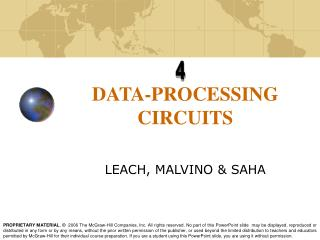DownloadDownload PresentationDATA-PROCESSING CIRCUITS

# DATA-PROCESSING CIRCUITS

Download Presentation## DATA-PROCESSING CIRCUITS

- - - - - - - - - - - - - - - - - - - - - - - - - - - E N D - - - - - - - - - - - - - - - - - - - - - - - - - - -
##### Presentation Transcript

1. 4 DATA-PROCESSING CIRCUITS LEACH, MALVINO & SAHA

2. Multiplexers • Multiplexer means many into one. A multiplexer is a circuit with many inputs but only one output. • The 74150 • Multiplexer Logic • Bubbles on Signal Lines • Universal logic circuit

3. Multiplexer • Nibble Multiplexer

4. Demultiplexers • Demultiplex means one into many. A demultiplexer is a logic circuit with one input and many outputs. • The 74154 (1-to-16 demultiplexer)

5. 1-OF-16 Decoder • A decoder is similar to a demultiplexer, with one exception—there is no data input. The only inputs are the control bits ABCD. This logic circuit is called 1-of-16 decoder because 1 of the 16 output line is high.

6. BCD-to-Decimal Decoder • BCD is an abbreviation for binary-coded decimal. The BCD code expresses each digit in a decimal number by its nibble equivalent. To anyone using BCD code, 0100 0010 1001 is equivalent to 429. Pinout diagram of 7445

7. Seven-Segment Decoder By forward biasing Seven LEDs labeled a through g, wee can display the digits 0 through 9. 7446 decoder driver 7448 decoder driver

8. Encoders • An encoder converts an active input signal into a coded output signal. • Decimal-To-BCD Encoder

9. Exclusive-OR Gates • The exclusive-OR gate has a high output only when an odd number of inputs is high. Y=AB+AB Logic symbol XOR gate Truth Table

10. Parity Generator and Checkers • Even parity means an n-bit input has an even numbers of 1s. For instances, 110011. Odd parity means an n-bit input has an odd number of 1s. For instance, 110001. • Parity Checker • Parity Generation • Application To check data transmission errors

11. MAGNITUDE COMPARATOR • Magnitude comparator compares magnitude two n-bit binary numbers, say X and Y and activates one of these three outputs X=Y, X>Y and X<Y. a) Block diagram of Magnitude comparator (b)Truth table and (c)circuit for 1-bit comparator

12. MAGNITUDE COMPARATOR a) Functional diagram of IC 7485 and (b) 8-bit comparator from two 4-bit comparators.

13. Read-only Memory • A read-only memory is an IC that can store thousands of binary numbers representing computer instructions and other fixed data. • DIODE ROM • Stores the binary numbers Diode ROM

14. Read-only Memory • On-Chip Decoding • Rather than switch-select the addresses as shown previously, a manufacturer uses on-chip decoding. With on-chip decoding, n inputs can select 2n memory locations.

15. Read-only Memory • Commercially Available ROMs • 7488: 256 bits organized as 32 x 8 • 74187: 1024 bits organized as 256 x 4 • 74S370: 2048 bits organized as 512 x 4 • Generating Boolean Functions • Programmable ROMs • A programmable ROM(PROM) allows the user instead of the manufacturer to store the data. • 74S188: 256 bits organized as 32 x 8 • 74S287: 1024 bits organized as 256 X 4 • 74S472: 4096 bits organized as 512 X 8

16. Read-only Memory • Simplified Drawing of a PROM • Programming a PROM • Generating a Boolean Function at the output of a PROM is accomplished by fusing fusible links at input to OR gates. Each X is a fusible link that can be removed.

17. Read-only Memory • Erasable PROMs • The erasable PROM (EPROM) uses metal-oxide-semiconductor field-effect transistors (MOSFETs). Data is stored with an EPROM programmer. Later, data can be erased with ultraviolet light. • Some of Commercially available EPROMs: • 2716: 16,384 bits organized as 2048 x 8 • 2732: 32,768 bits organized as 4096 x 8 • The EPROM is useful in project development. With an EPROM, the designer can modify the content until the stored data is perfect.

18. Programmable Array Logic • PAL is a programmable array of logic gates on a single chip.

19. Programmable Array Logic • Programming A PAL • A PAL is different from a PROM because it has a programmable AND array and a fixed OR array. Y3=ABCD+ABCD+ABCD+ABCD Y2=ABCD+ABCD+ABCD Y1=ABC+ABC+ABC+ABC Y0=ABCD

20. Programmable Array Logic • Commercially available PALs • 10H8: 10 input and 8 output AND-OR • 16H2: 6 input and 2 output AND-OR • 14L4: 14 input and 4 output AND-OR-INVERT For these chip numbers, H stands for active-high output and L for active-low output.

21. Programmable logic Arrays • PLAs, along with ROMs and PALs, are included in more general classification of ICs called programmable logicdevices(PLDs). PAL PLA

22. HDL implementation of data processing circuits • Hardware design of multiplexers using Verilog code. The behavioral model can be used to describe the 2 to 1 multiplexers in following different ways

23. Bus representation in HDL • The Verilog code for demultiplexer/decoder.